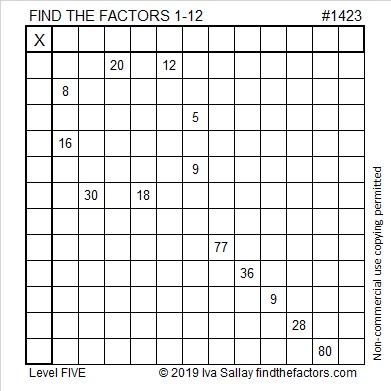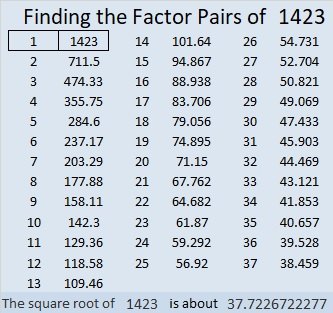# 1423 Lollipop

Lollipops are candies that easily delight children. Will today’s lollipop-wannabe puzzle be a delight?Print the puzzles or type the solution in this excel file: 12 Factors 1419-1429

The puzzle number is 1423. Here are a few facts about that number:

• 1423 is a prime number.
• Prime factorization: 1423 is prime.
• 1423 has no exponents greater than 1 in its prime factorization, so √1423 cannot be simplified.
• The exponent in the prime factorization is 1. Adding one to that exponent we get (1 + 1) = 2. Therefore 1423 has exactly 2 factors.
• The factors of 1423 are outlined with their factor pair partners in the graphic below.How do we know that 1423 is a prime number? If 1423 were not a prime number, then it would be divisible by at least one prime number less than or equal to √1423. Since 1423 cannot be divided evenly by 2, 3, 5, 7, 11, 13, 17, 19, 23, 29, 31, or 37, we know that 1423 is a prime number.

This site uses Akismet to reduce spam. Learn how your comment data is processed.